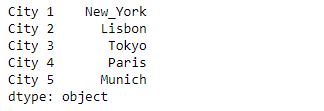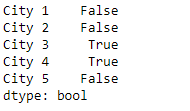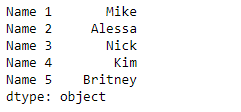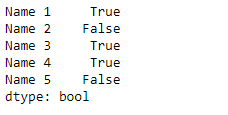# Python | Pandas Series.str.match()

`Series.str` can be used to access the values of the series as strings and apply several methods to it. Pandas` Series.str.match()` function is used to determine if each string in the underlying data of the given series object matches a regular expression.

Syntax: Series.str.match(pat, case=True, flags=0, na=nan)

Parameter :
pat : Regular expression pattern with capturing groups.
case : If True, case sensitive
flags : A re module flag, for example re.IGNORECASE.
na : default NaN, fill value for missing values

Returns : Series/array of boolean values

Example #1: Use `Series.str.match()` function to match the passed regular expressions with the string in the underlying data of the given series object.

 `# importing pandas as pd ` `import` `pandas as pd ` ` `  `# importing re for regular expressions ` `import` `re ` ` `  `# Creating the Series ` `sr ``=` `pd.Series([``'New_York'``, ``'Lisbon'``, ``'Tokyo'``, ``'Paris'``, ``'Munich'``]) ` ` `  `# Creating the index ` `idx ``=` `[``'City 1'``, ``'City 2'``, ``'City 3'``, ``'City 4'``, ``'City 5'``] ` ` `  `# set the index ` `sr.index ``=` `idx ` ` `  `# Print the series ` `print``(sr) `

Output :Now we will use `Series.str.match()` function to match the passed regular expressions with the string in the underlying data of the given series object.

 `# match either 'Tokyo' or 'Paris' ` `result ``=` `sr.``str``.match(pat ``=` `'(Tokyo)|(Paris)'``) ` ` `  `# print the result ` `print``(result) `

Output :As we can see in the output, the `Series.str.match()` function has returned a series of boolean values. It contains `True` for those values which successfully matches else it contains `False`.

Example #2 : Use `Series.str.match()` function to match the passed regular expressions with the string in the underlying data of the given series object.

 `# importing pandas as pd ` `import` `pandas as pd ` ` `  `# importing re for regular expressions ` `import` `re ` ` `  `# Creating the Series ` `sr ``=` `pd.Series([``'Mike'``, ``'Alessa'``, ``'Nick'``, ``'Kim'``, ``'Britney'``]) ` ` `  `# Creating the index ` `idx ``=` `[``'Name 1'``, ``'Name 2'``, ``'Name 3'``, ``'Name 4'``, ``'Name 5'``] ` ` `  `# set the index ` `sr.index ``=` `idx ` ` `  `# Print the series ` `print``(sr) `

Output :Now we will use `Series.str.match()` function to match the passed regular expressions with the string in the underlying data of the given series object.

 `# match groups having any capital letter ` `# followed by 'i' and any other character ` `result ``=` `sr.``str``.match(pat ``=` `'([A-Z]i.)'``) ` ` `  `# print the result ` `print``(result) `

Output :As we can see in the output, the `Series.str.match()` function has returned a series of boolean values. It contains `True` for those values which successfully matches else it contains `False`.

My Personal Notes arrow_drop_upCheck out this Author's contributed articles.

If you like GeeksforGeeks and would like to contribute, you can also write an article using contribute.geeksforgeeks.org or mail your article to contribute@geeksforgeeks.org. See your article appearing on the GeeksforGeeks main page and help other Geeks.

Please Improve this article if you find anything incorrect by clicking on the "Improve Article" button below.

Article Tags :

Be the First to upvote.

Please write to us at contribute@geeksforgeeks.org to report any issue with the above content.